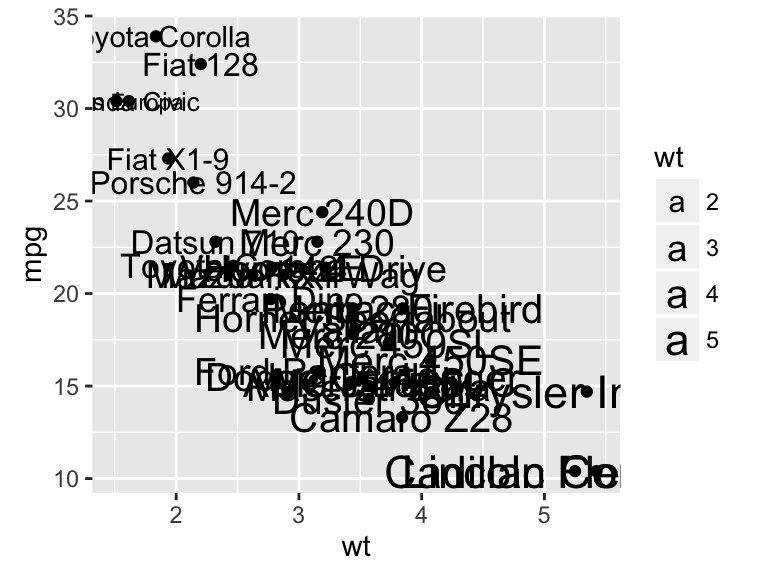ggplot2 texts : Add text annotations to a graph in R software

The functions below can be used :

• geom_text(): adds text directly to the plot
• geom_label(): draws a rectangle underneath the text, making it easier to read.
• annotate(): useful for adding small text annotations at a particular location on the plot
• annotation_custom(): Adds static annotations that are the same in every panel

It’s also possible to use the R package ggrepel, which is an extension and provides geom for ggplot2 to repel overlapping text labels away from each other.

We’ll start by describing how to use ggplot2 official functions for adding text annotations. In the last sections, examples using ggrepel extensions are provided.

Install required packages

# Install ggplot2
install.packages("ggplot2")
# Install ggrepel
install.packages("ggrepel")

Create some data

We’ll use a subset of mtcars data. The function sample() can be used to randomly extract 10 rows:

# Subset 10 rows
set.seed(1234)
ss <- sample(1:32, 10)
df <- mtcars[ss, ]

Text annotations using geom_text and geom_label

library(ggplot2)
# Simple scatter plot
sp <- ggplot(df, aes(wt, mpg, label = rownames(df)))+
geom_point()

sp + geom_text()
# Change the size of the texts
sp + geom_text(size=6)
# Change vertical and horizontal adjustement
sp +  geom_text(hjust=0, vjust=0)
# Change fontface. Allowed values : 1(normal),
# 2(bold), 3(italic), 4(bold.italic)
sp + geom_text(aes(fontface=2))• Change font family
sp + geom_text(family = "Times New Roman")
• geom_label() works like geom_text() but draws a rounded rectangle underneath each label. This is useful when you want to label plots that are dense with data.
sp + geom_label()Others useful arguments for geom_text() and geom_label() are:

• nudge_x and nudge_y: let you offset labels from their corresponding points. The function position_nudge() can be also used.
• check_overlap = TRUE: for avoiding overplotting of labels
• hjust and vjust can now be character vectors (ggplot2 v >= 2.0.0): “left”, “center”, “right”, “bottom”, “middle”, “top”. New options include “inward” and “outward” which align text towards and away from the center of the plot respectively.

Change the text color and size by groups

It’s possible to change the appearance of the texts using aesthetics (color, size,…) :

sp2 <- ggplot(mtcars, aes(x=wt, y=mpg, label=rownames(mtcars)))+
geom_point()
# Color by groups
sp2 + geom_text(aes(color=factor(cyl)))# Set the size of the text using a continuous variable
sp2 + geom_text(aes(size=wt))# Define size range
sp2 + geom_text(aes(size=wt)) + scale_size(range=c(3,6))Add a text annotation at a particular coordinate

The functions geom_text() and annotate() can be used :

# Solution 1
sp2 + geom_text(x=3, y=30, label="Scatter plot")
# Solution 2
sp2 + annotate(geom="text", x=3, y=30, label="Scatter plot",
color="red")annotation_custom : Add a static text annotation in the top-right, top-left, …

The functions annotation_custom() and textGrob() are used to add static annotations which are the same in every panel.The grid package is required :

library(grid)
# Create a text
grob <- grobTree(textGrob("Scatter plot", x=0.1,  y=0.95, hjust=0,
gp=gpar(col="red", fontsize=13, fontface="italic")))
# Plot
sp2 + annotation_custom(grob)Facet : In the plot below, the annotation is at the same place (in each facet) even if the axis scales vary.

sp2 + annotation_custom(grob)+facet_wrap(~cyl, scales="free")ggrepel: Avoid overlapping of text labels

There are two important functions in ggrepel R packages:

• geom_label_repel()
• geom_text_repel()

Scatter plots with text annotations

We start by creating a simple scatter plot using a subset of the mtcars data set containing 15 rows.

1. Prepare some data:
# Take a subset of 15 random points
set.seed(1234)
ss <- sample(1:32, 15)
df <- mtcars[ss, ]
1. Create a scatter plot:
p <- ggplot(df, aes(wt, mpg)) +
geom_point(color = 'red') +
theme_classic(base_size = 10)
# Add text annotations using ggplot2::geom_text
p + geom_text(aes(label = rownames(df)),
size = 3.5)# Use ggrepel::geom_text_repel
require("ggrepel")
set.seed(42)
p + geom_text_repel(aes(label = rownames(df)),
size = 3.5)# Use ggrepel::geom_label_repel and
# Change color by groups
set.seed(42)
p + geom_label_repel(aes(label = rownames(df),
fill = factor(cyl)), color = 'white',
size = 3.5) +
theme(legend.position = "bottom")Volcano plot

genes\$Significant <- ifelse(genes\$padj < 0.05, "FDR < 0.05", "Not Sig")
ggplot(genes, aes(x = log2FoldChange, y = -log10(pvalue))) +
geom_point(aes(color = Significant)) +
scale_color_manual(values = c("red", "grey")) +
theme_bw(base_size = 12) + theme(legend.position = "bottom") +
geom_text_repel(
data = subset(genes, padj < 0.05),
aes(label = Gene),
size = 5,
)source

Infos

This analysis has been performed using R software (ver. 3.2.4) and ggplot2 (ver. )